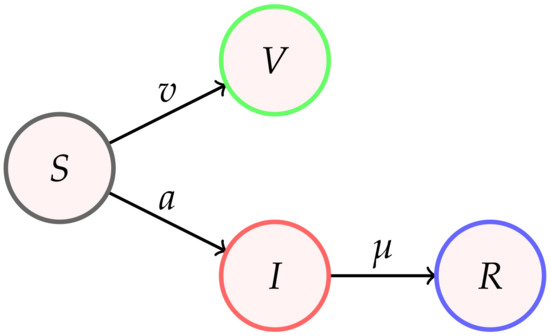Next Article in Journal
Spin Current in BF Theory
Next Article in Special Issue
SIR-PID: A Proportional–Integral–Derivative Controller for COVID-19 Outbreak Containment
Previous Article in Journal
Dynamics of a Homogeneous and Isotropic Space in Pure Cubic f(R) Gravity
Previous Article in Special Issue
Comparison of Main Covid-19 Outbreaks and Interpretation Based on Age Differences

Article

# Analytical Modeling of the Temporal Evolution of Epidemics Outbreaks Accounting for Vaccinations

1
Institut für Theoretische Physik, Lehrstuhl IV: Weltraum-Und Astrophysik, Ruhr-Universität Bochum, D-44780 Bochum, Germany
2
Institut für Theoretische Physik und Astrophysik, Christian-Albrechts-Universität zu Kiel, Leibnizstr. 15, D-24118 Kiel, Germany
3
Polymer Physics, Department of Materials, ETH Zurich, CH-8093 Zurich, Switzerland
*
Authors to whom correspondence should be addressed.
Physics 2021, 3(2), 386-426; https://doi.org/10.3390/physics3020028
Received: 28 March 2021 / Revised: 29 April 2021 / Accepted: 12 May 2021 / Published: 25 May 2021
(This article belongs to the Special Issue Physics Methods in Coronavirus Pandemic Analysis)
With the vaccination against Covid-19 now available, how vaccination campaigns influence the mathematical modeling of epidemics is quantitatively explored. In this paper, the standard susceptible-infectious-recovered/removed (SIR) epidemic model is extended to a fourth compartment, V, of vaccinated persons. This extension involves the time t-dependent effective vaccination rate, $v\left(t\right)$, that regulates the relationship between susceptible and vaccinated persons. The rate $v\left(t\right)$ competes with the usual infection, $a\left(t\right)$, and recovery, $\mu \left(t\right)$, rates in determining the time evolution of epidemics. The occurrence of a pandemic outburst with rising rates of new infections requires $k+b<1-2\eta$, where $k=\mu \left(0\right)/a\left(0\right)$ and $b=v\left(0\right)/a\left(0\right)$ denote the initial values for the ratios of the three rates, respectively, and $\eta \ll 1$ is the initial fraction of infected persons. Exact analytical inverse solutions $t\left(Q\right)$ for all relevant quantities $Q=\left[S,I,R,V\right]$ of the resulting SIRV model in terms of Lambert functions are derived for the semi-time case with time-independent ratios k and b between the recovery and vaccination rates to the infection rate, respectively. These inverse solutions can be approximated with high accuracy, yielding the explicit time-dependences $Q\left(t\right)$ by inverting the Lambert functions. The values of the three parameters k, b and $\eta$ completely determine the reduced time evolution of the SIRV-quantities $Q\left(\tau \right)$. The influence of vaccinations on the total cumulative number and the maximum rate of new infections in different countries is calculated by comparing with monitored real time Covid-19 data. The reduction in the final cumulative fraction of infected persons and in the maximum daily rate of new infections is quantitatively determined by using the actual pandemic parameters in different countries. Moreover, a new criterion is developed that decides on the occurrence of future Covid-19 waves in these countries. Apart from in Israel, this can happen in all countries considered. View Full-Text
Show FiguresFigure 1

MDPI and ACS Style

Schlickeiser, R.; Kröger, M. Analytical Modeling of the Temporal Evolution of Epidemics Outbreaks Accounting for Vaccinations. Physics 2021, 3, 386-426. https://doi.org/10.3390/physics3020028

AMA Style

Schlickeiser R, Kröger M. Analytical Modeling of the Temporal Evolution of Epidemics Outbreaks Accounting for Vaccinations. Physics. 2021; 3(2):386-426. https://doi.org/10.3390/physics3020028

Chicago/Turabian Style

Schlickeiser, Reinhard, and Martin Kröger. 2021. "Analytical Modeling of the Temporal Evolution of Epidemics Outbreaks Accounting for Vaccinations" Physics 3, no. 2: 386-426. https://doi.org/10.3390/physics3020028

Find Other Styles

1